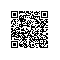# C语言分解数组

[程序说明]

4 = 4

4 = 3 +1

4 = 2 +2

4 = 2 +1 +1

4 = 1 + 1 + 1 + 1

1 #define MAXN 100
2  int a[MAXN],r[MAXN];
3 rd(int n,int k)
4 { int j,i;
5 for (j=n<a[k-1]?n:a[k-1];j>=1;j--)
6 {
7 a[k]=j;
8 if (j==n)
9 {
10 printf("％d = ％d",a,a);
11 for (i=2;i<=k;i++)
12 printf("+％d",a[i]);
13 printf("\n");
14 }
15 else rd(n-j,k+1);
16 }
17 }
18
19 nd (int n)
20 {
21 int i,k;
22 k=0;
23 r=n;
24 do
25 {
26 if (r[k]==0)
27 {
28 printf("％d=％d",a,a);
29 for (i=2;i<=k;i++)
30 printf("+％d",a[i]);
31 printf("\n");
32 while (k>0 && a[k]==1) k--;
33 if (k>0) { a[k]--;r[k]++;}
34 }
35 else
36 {
37 a[k+1]=a[k]<r[k]?a[k]:r[k];
38 r[k+1]=r[k]-a[k+1];k++;
39 }
40 } while (k>0);
41 };
42
43 int test_data[]={3,4,5};
44 main()
45 {
46 int i;
47 for (i=0;i<sizeof(test_data)/sizeof(int);i++)
48 {
49 a=test_data[i];
50 rd(test_data[i],1);
51 printf("\n__________\n\n");
52 nd(test_data[i]);
53 printf( "\n_________\n\n");
54 }
55 }使用钉钉扫一扫加入圈子
+ 订阅